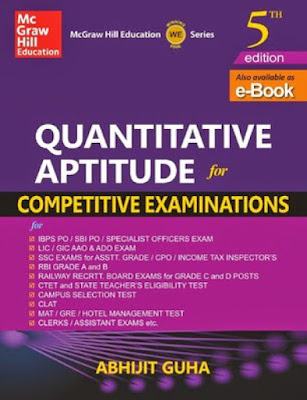## Content In Abhijit Guha Quantitative Aptitude Free Ebook In PDF1. Number System
2. Number Series
3. HCF and LCM of Numbers
4. Fractions and Decimals
5. Square Root and Cube Roots
6. Indices and Surds
7. Vedic Mathematics
8. Simplification and Approximation
9. Problems on Ages and Numbers
Exam Level Test – 1
10. Percentage
11. Profit, Loss and Discount
12. Average
13. Ratio and Proportion
14. Partnership and Share
15. Alligation and Mixtures
Exam Level Test – 2
16. Time, Work and Wages
17. Pipes and Cisterns
18. Simple Interest
19. Compound Interest, Growth and Depreciation
Exam Level Test – 3
20. Time and Distance
21. Trains
22. Boats and Streams
23. Races
24. Clocks
25. Calendar
Exam Level Test – 4
26. Area of Plane Figures
27. Volume and Surface Area of Solid Figures
28. Elementry Algebra
29. Linear Equations
31. Progression quantitative Aptitude PDF
Exam Level Test – 5
32. Permutation and Combination
33. Probability
34. Geometry
35. Trigonometry
Exam Level Test – 6
36. Data Interpretation
37. Data Sufficiency
Mock Test Papers
Latest Exam Papers

Quantitative Aptitude Study Materials PDF

How to Join bank4success Telegram Group for Discussion